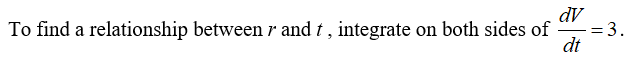# 3.6.29-GIHelium is pumped into a spherical balloon at a constant rate of 3 cubic feet per second How fast is the radius increasing after 4 minutes? At what time (if any) is the radius increasing at a rate of 120 feet per second?The radius is increasing at a rate of(Type an integer or a decimal. Do not round until the final answer. Then round to four decimal places as needed.)ft/sec.Question ViewerEnter your answer in the answer box and then click Check Answer.Clear Allpartremaining1O HiType here to searchhpt aftvofofen*20044

Question
96 views
check_circle

Step 1

Helium is pumped into spherical balloon at a rate of 3 cubic feet per second.

Step 2

Consider the equation

Step 3...

### Want to see the full answer?

See Solution

#### Want to see this answer and more?

Solutions are written by subject experts who are available 24/7. Questions are typically answered within 1 hour.*

See Solution
*Response times may vary by subject and question.
Tagged in

### Other